# CHAR Function In ExcelArticle byExcelMojo Team## What Is CHAR Function In Excel?

The CHAR function in Excel is an inbuilt Text function that returns the character specified by the given number. Users can utilize the CHAR() when translating code page numbers obtained from files on different computer types into characters. The function is also useful for inserting a line break into the given text value.

For example, the table below shows a data set and the requirement description for displaying each data value.

We have to show the output in column C. So, we can achieve our desired results using CHAR function in Excel in the target cells.

The above table explains the CHAR function with example scenarios. Not only we can display a given number specifically by applying the CHAR(), but we can also use it to insert characters into a given data according to our requirements.

###### Key Takeaways
• The CHAR function in Excel determines the character specified by the given number. Users can apply this function for converting code page numbers in files received from other computer systems into characters.
• The CHAR() accepts one argument, number, as input.
• The character that the CHAR() returns for the given character code (argument number) depends on our computer OS’s character set. For example, while the character set for WINDOWS OS is ANSI, it is Macintosh for MAC OS.
• The CHAR() gives excellent results when we use it with other Excel functions such as SUBSTITUTE and CODE.

### CHAR() Excel Formula

The CHAR excel formula is:

where,

• number: A number specifying the character we require from the character set defined in our computer’s operating system. The value of this argument can range between 1 and 255.

The number argument in the CHAR excel formula is mandatory. We can directly select the specific number or provide the excel cell reference to the argument.

Please Note: The CHAR function in Excel output depends on the user’s computer OS, as the character set varies with each OS. For example, WINDOWS OS utilizes the ANSI character set, while the MAC OS works with the Macintosh character set.

Below are a few crucial aspects of the CHAR function we must know to use it properly.

• The CHAR() accepts only one number as input. Therefore, the function will not work if we select multiple values separated by a comma in the argument. However, we can enter a continuous cell range as the function argument and execute the CHAR() as an excel array formula using the keys Ctrl + Shift + Enter.
• Suppose we provide the number argument as 0 or an integer not between 1 and 255 to the CHAR(). Then the CHAR function return value will be the #VALUE! error.
• The CHAR() output is a string or text value.
• The CHAR() is the inverse of the Excel CODE(), which returns the code for a specified character.

### How To Use CHAR Excel Function?

Here is how we can utilize the CHAR function in Excel:

1. First, ensure that the number we provide as an input to the CHAR() is between 1 and 255
2. Then, select the target cell and enter the CHAR().
3. Finally, press Enter to view the result as a text or string value.

Let us see these steps to apply the CHAR function in Excel with example.

The following table contains two strings, Text 1 and Text 2, in columns A and B.

And suppose we require to add the two given strings with a specific character in between, mentioned in column C. We can do so using CHAR function in Excel, with the corresponding character code as the input to the CHAR() in the formula.

Step 1: Select the target cell E2, enter the below formula containing the CHAR(), and press Enter.

=A2&CHAR(D2)&B2

Alternatively, we can select the target cell E2, enter ‘=’, cell reference A2, ‘&’, and click FormulasTextCHAR to open the Function Arguments window.

Then enter argument number D2, the cell reference to character code 45, in the Function Arguments window.

Once we click OK in the Function Arguments window, the target cell value will be:

Finally, complete the formula by entering ‘&’ and cell reference B2.

And once we press Enter, the output will be as depicted earlier.

Step 2: Drag the fill handle downwards to copy the formula in the range E3:E6.

In the above example, the formula displays the first text, and then the CHAR function in Excel output is the character the number specifies. And finally, the formula displays the second text to show the final text, as per the requirement.

### Examples

To understand it effectively, let us see some more CHAR function in Excel examples.

#### Example #1

The below illustration explains how we can utilize the various special characters that Excel offers in our daily tasks by applying the CHAR function.

Consider the below table. It shows different sections of a text, their respective values, and the symbols to add in each case, as explained in column C.

We can get the desired results using the code corresponding to the required character in the CHAR function in Excel in each target cell in column D.

Step 1: Select the target cell D2, enter the following CHAR(), and press Enter.

=CHAR(149)&” “&B2

Step 2: Likewise, enter the formulas containing the CHAR() with the argument number being the required character code, as depicted in the below image.

So, once we execute the above formulas in the cell range D3:D6, the result will be:

Thus we can apply the CHAR() with the ‘&’ to append the required characters in a string or text value. But we must know the respective character code to provide input to the CHAR() we use in each target cell. Also, we need to place the function in the formula, where we require the symbol to appear in the final text.

#### Example #2

This example shows how the CHAR function helps add string values with line breaks.

Consider the following table containing customers’ address details in columns B:E.

Suppose we must add the four strings in columns B:E and display them in the respective target cells in column F, with line breaks inserted between the strings. Then we can apply the CHAR function in Excel to make the process straightforward.

Step 1: Select the target cell F2, enter the below formula, and press Enter.

=B2&CHAR(10)&C2&CHAR(10)&D2&CHAR(10)&E2

The number argument in the CHAR() is 10, which specifies a line break. Thus, the above formula inserts line breaks between the given strings while adding them.

Step 2: Select cells F2 and click HomeWrap Text.

Wrapping the text will make the line breaks visible in the CHAR function in Excel return value.

Step 3: Drag the fill handle downwards to copy the formula in the range F3:F6.

Thus, the CHAR() avoids manually adding the required line breaks in the Formula Bar.

#### Example #3

We can apply the CHAR function with functions such as SUBSTITUTE and CODE Function in Excel.

Assume the below table contains a list of phone numbers in one format.

And we require to remove the hyphens in each phone number to display the numbers in another format.

We can apply the CHAR function with CODE and SUBSTITUTE Function in Excel to achieve the desired results.

Step 1: Select the target cell B2, enter the below formula containing the CHAR, SUBSTITUTE, and CODE functions, and press Enter.

=SUBSTITUTE(A2,CHAR(CODE(“-“)),””)

Step 2: Drag the fill handle downwards to copy the formula in range B3:B7.

Let us see how the above formula works. Consider the expression in the target cell B7. First, the CODE() returns the character code of ‘‘, 45. Next, the CHAR() returns the character specified by the number argument 45, ‘‘. And finally, the SUBSTITUTE() replaces all instances of the symbol, ‘‘, with a blank.

### Important Things To Note

• The CHAR function in Excel returns a string or text value.
• We can enter the CHAR() argument directly as the specified number or cell reference to the number. And suppose we enter the function argument as a cell range reference to the set of given character codes. Then we must execute the CHAR() as an array formula using Ctrl + Shift + Enter.
• And we can utilize the CHAR() to add a line break in the given data.
• If the argument number is not between 1 and 255, the CHAR() return value will be the #VALUE! error.

1. Where is the CHAR function in Excel?

The CHAR function in Excel is in the Formulas tab. Click FormulasText CHAR to access it.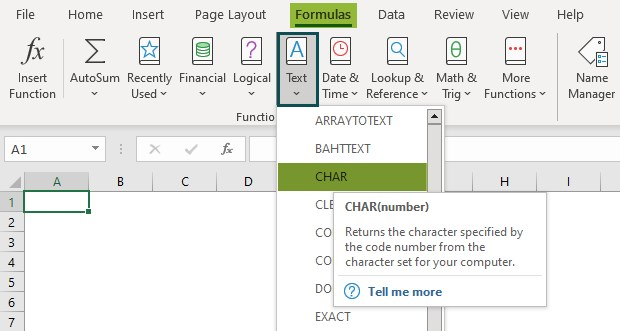2. How do I find a CHAR number for the CHAR function in Excel?

We can find a CHAR number for the CHAR function using the CODE().
For example, the table below contains a list of characters.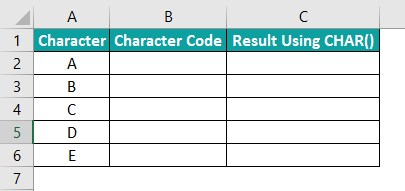Suppose we have to determine the character code for each character in cells A2:A6 and display them in column B cells B2:B6. And these character codes will be the number argument in the CHAR() formulas we will use in cells C2:C6. Then, the steps to complete the required actions are:

Step 1: Select cell B2, enter the CODE() provided in the Formula Bar in the image below, and press Enter.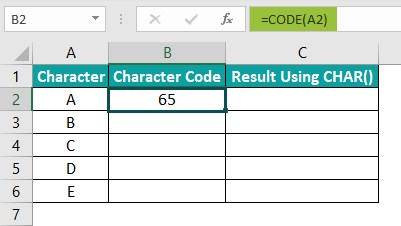Step 2: Drag the fill handle downwards to copy the formula in the cell range B3:B6.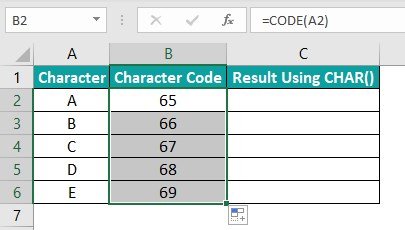Step 3: Select cell C2, enter the CHAR() provided in the Formula Bar in the below image, and press Enter.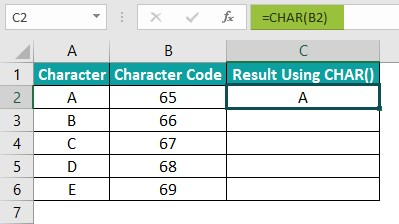Step 4: Drag the fill handle downwards to copy the formula in range C3:C6.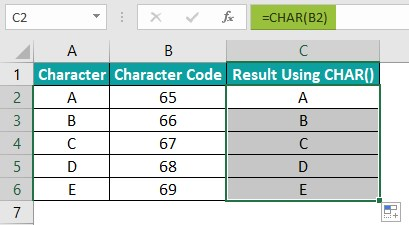3. How do I replace CHAR 160 in Excel?

We can replace CHAR 160 in excel using the below steps. Let us see them with an example.

Assume the below table shows area ZIP codes. But the source data contains non-breaking spaces.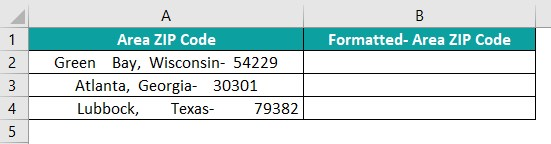And we require to display the data in a proper format in the target cell range B2:B4, without the non-breaking spaces.

We can achieve the desired results using the CHAR() with TRIM and SUBSTITUTE functions, and the steps are:

Step 1: Select the target cell B2, enter the formula provided in the Formula Bar in the below image, and press Enter key.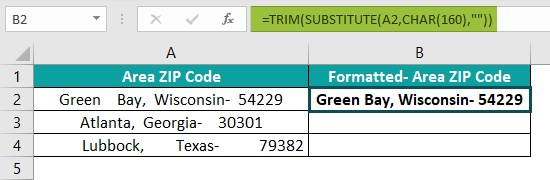Step 2: Drag the fill handle downwards to copy the formula in the range B3:B4.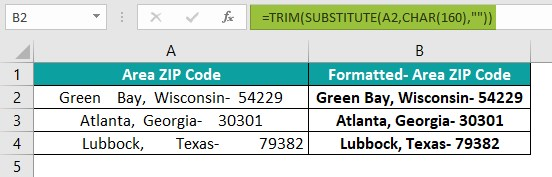The character code for a non-breaking space in the ANSI character set is 160. So, first, the CHAR(160) in each formula in cells B2:B4 returns a non-breaking space. Then the SUBSTITUTE() replaces each non-breaking space with a regular space. And finally, the TRIM() removes all the spaces in the text value, leaving the single spaces between the words to return the text value according to the requirement.